Discrete Mathematics and Combinatorics Commons™

968 Full-Text Articles 1,127 Authors 104,022 Downloads94 Institutions

All Articles in Discrete Mathematics and Combinatorics

968 full-text articles. Page 9 of 36.

Zero Forcing And Maximum Nullity For Hypergraphs, 2018Iowa State University and American Institute of Mathematics

Zero Forcing And Maximum Nullity For Hypergraphs, Leslie Hogben

Mathematics Publications

The concept of zero forcing is extended from graphs to uniform hypergraphs in analogy with the way zero forcing was defined as an upper bound for the maximum nullity of the family of symmetric matrices whose nonzero pattern of entries is described by a given graph: A family of symmetric hypermatrices is associated with a uniform hypergraph and zeros are forced in a null vector. The value of the hypergraph zero forcing number and maximum nullity are determined for various families of uniform hypergraphs and the effects of several graph operations on the hypergraph zero forcing number are determined. The ...

Graphs That Are Cospectral For The Distance Laplacian, 2018Rice University

Graphs That Are Cospectral For The Distance Laplacian, Boris Brimkov, Ken Duna, Leslie Hogben, Kate Lorenzen, Carolyn Reinhart, Sung-Yell Song, Mark Yarrow

Mathematics Publications

The distance matrix D(G) of a graph G is the matrix containing the pairwise distances between vertices, and the distance Laplacian matrix is DL(G)=T(G)−D(G), where T(G) is the diagonal matrix of row sums of D(G). We establish several general methods for producing DL-cospectral graphs that can be used to construct infinite families. We provide examples showing that various properties are not preserved by DL-cospectrality, including examples of DL-cospectral strongly regular and circulant graphs. We establish that the absolute values of coefficients of the distance Laplacian characteristic polynomial ...

Rigid Linkages And Partial Zero Forcing, 2018Texas State University - San Marcos

Rigid Linkages And Partial Zero Forcing, Daniela Ferrero, Mary Flagg, H. Tracy Hall, Leslie Hogben, Jephian C.-H. Lin, Seth A. Meyer, Shahla Nasserasr, Bryan Shader

Mathematics Publications

Connections between vital linkages and zero forcing are established. Specifically, the notion of a rigid linkage is introduced as a special kind of unique linkage and it is shown that spanning forcing paths of a zero forcing process form a spanning rigid linkage and thus a vital linkage. A related generalization of zero forcing that produces a rigid linkage via a coloring process is developed. One of the motivations for introducing zero forcing is to provide an upper bound on the maximum multiplicity of an eigenvalue among the real symmetric matrices described by a graph. Rigid linkages and a related ...

The Relationship Between K-Forcing And K-Power Domination, 2018Texas State University

The Relationship Between K-Forcing And K-Power Domination, Daniela Ferrero, Leslie Hogben, Franklin H.J. Kenter, Michael Young

Mathematics Publications

Zero forcing and power domination are iterative processes on graphs where an initial set of vertices are observed, and additional vertices become observed based on some rules. In both cases, the goal is to eventually observe the entire graph using the fewest number of initial vertices. The concept of k-power domination was introduced by Chang et al. (2012) as a generalization of power domination and standard graph domination. Independently, k-forcing was defined by Amos et al. (2015) to generalize zero forcing. In this paper, we combine the study of k-forcing and k-power domination, providing a new approach to analyze both ...

Applications Of Analysis To The Determination Of The Minimum Number Of Distinct Eigenvalues Of A Graph, 2018Iowa State University

Applications Of Analysis To The Determination Of The Minimum Number Of Distinct Eigenvalues Of A Graph, Beth Bjorkman, Leslie Hogben, Scarlitte Ponce, Carolyn Reinhart, Theodore Tranel

Mathematics Publications

We establish new bounds on the minimum number of distinct eigenvalues among real symmetric matrices with nonzero off-diagonal pattern described by the edges of a graph and apply these to determine the minimum number of distinct eigenvalues of several families of graphs and small graphs.

On The Strong Chromatic Index Of Sparse Graphs, 2018Westfield State University

On The Strong Chromatic Index Of Sparse Graphs, Philip Deorsey, Michael Ferrara, Nathan Graber, Stephen G. Hartke, Luke L. Nelsen, Eric Sullivan, Sogol Jahanbekam, Bernard Lidicky, Derrick Stolee, Jennifer White

Mathematics Publications

The strong chromatic index of a graph G, denoted χ′s(G), is the least number of colors needed to edge-color G so that edges at distance at most two receive distinct colors. The strong list chromatic index, denoted χ′s,ℓ(G), is the least integer k such that if arbitrary lists of size k are assigned to each edge then G can be edge-colored from those lists where edges at distance at most two receive distinct colors.

We use the discharging method, the Combinatorial Nullstellensatz, and computation to show that if G is a subcubic planar graph with ...

Self-Assembly Of Dna Graphs And Postman Tours, 2018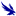University of North Florida

Self-Assembly Of Dna Graphs And Postman Tours, Katie Bakewell

DNA graph structures can self-assemble from branched junction molecules to yield solutions to computational problems. Self-assembly of graphs have previously been shown to give polynomial time solutions to hard computational problems such as 3-SAT and k-colorability problems. Jonoska et al. have proposed studying self-assembly of graphs topologically, considering the boundary components of their thickened graphs, which allows for reading the solutions to computational problems through reporter strands. We discuss weighting algorithms and consider applications of self-assembly of graphs and the boundary components of their thickened graphs to problems involving minimal weight Eulerian walks such as the Chinese Postman Problem and ...

2018Georgia Southern University

Sparse Trees With A Given Degree Sequence, Ao Shen

Electronic Theses and Dissertations

In this thesis, we consider the properties of sparse trees and summarized a certain class of trees under some constraint (including with a given degree sequence, with given number of leaves, with given maximum degree, etc.) which have maximum Wiener index and the minimum number of subtrees at the same time. Wiener index is one of the most important topological indices in chemical graph theory. Steiner k􀀀 Wiener index can be regarded as the generalization of Wiener index, when k = 2, Steiner Wiener index is the same as Wiener index. Steiner k􀀀 Wiener index of a tree T is the ...

On Saturation Numbers Of Ramsey-Minimal Graphs, 2018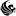University of Central Florida

On Saturation Numbers Of Ramsey-Minimal Graphs, Hunter M. Davenport

Dating back to the 1930's, Ramsey theory still intrigues many who study combinatorics. Roughly put, it makes the profound assertion that complete disorder is impossible. One view of this problem is in edge-colorings of complete graphs. For forbidden graphs H1,...,Hk and a graph G, we write G "arrows" (H1,...,Hk) if every k-edge-coloring of G contains a monochromatic copy of Hi in color i for some i=1,2,...,k. If c is a (red, blue)-edge-coloring of G, we say c is a bad coloring if G contains no red K3or ...

On The Planarity Of Generalized Line Graphs, 2018Western Michigan University

On The Planarity Of Generalized Line Graphs, Khawlah H. Alhulwah, Mohra Zayed, Ping Zhang

Theory and Applications of Graphs

One of the most familiar derived graphs is the line graph. The line graph $L(G)$ of a graph $G$ is that graph whose vertices are the edges of $G$ where two vertices of $L(G)$ are adjacent if the corresponding edges are adjacent in~$G$. Two nontrivial paths $P$ and $Q$ in a graph $G$ are said to be adjacent paths in $G$ if $P$ and $Q$ have exactly one vertex in common and this vertex is an end-vertex of both $P$ and $Q$. For an integer $\ell \ge 2$, the $\ell$-line graph $L_{\ell}(G)$ of a ...

An Incidence Approach To The Distinct Distances Problem, 2018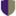Claremont Colleges

An Incidence Approach To The Distinct Distances Problem, Bryce Mclaughlin

In 1946, Erdös posed the distinct distances problem, which asks for the minimum number of distinct distances that any set of n points in the real plane must realize. Erdös showed that any point set must realize at least &Omega(n1/2) distances, but could only provide a construction which offered &Omega(n/&radic(log(n)))$distances. He conjectured that the actual minimum number of distances was &Omega(n1-&epsilon) for any &epsilon > 0, but that sublinear constructions were possible. This lower bound has been improved over the years, but Erdös' conjecture seemed to hold until in 2010 ... 2018University of Colorado, Boulder Finding Planted Cliques In Erdős–Rényi Random Graphs: Improving Previous Methods And Expanding Applications, Megan Sochinski Undergraduate Honors Theses In this paper, we will discuss new methods for finding planted cliques within Erdős–Rényi random graphs. An Erdős–Rényi random graph is a graph with n vertices, where each vertex is connected to each other vertex with some probability p, independent of all other choices. The planted clique problem asks us to find the most efficient way to find a planted clique in an Erdős–Rényi random graph. A planted clique is a secretly chosen set of vertices in the graph that are purposefully connected with edges added to the graph until all of the selected vertices are connected ... Lights Out! On Cartesian Products, 2017Iowa State University Lights Out! On Cartesian Products, Travis Peters, John Goldwasser, Michael Young Electronic Journal of Linear Algebra The game LIGHTS OUT! is played on a 5 by 5 square grid of buttons; each button may be on or off. Pressing a button changes the on/o state of the light of the button pressed and of all its vertical and horizontal neighbors. Given an initial configuration of buttons that are on, the object of the game is to turn all the lights out. The game can be generalized to arbitrary graphs. In this paper, Cartesian Product graphs (that is, graphs of the form G\box H, where G and H are arbitrary finite, simple graphs) are investigated ... Upper Bounds On Q-Spectral Radius Of Book-Free And/Or$K_{S,T}$-Free Graphs, 2017Northwestern Polytechnical University Upper Bounds On Q-Spectral Radius Of Book-Free And/Or$K_{S,T}$-Free Graphs, Qi Kong, Ligong Wang Electronic Journal of Linear Algebra In this paper, we prove two results about the signless Laplacian spectral radius$q(G)$of a graph$G$of order$n$with maximum degree$\Delta$. Let$B_{n}=K_{2}+\overline{K_{n}}$denote a book, i.e., the graph$B_{n}$consists of$n$triangles sharing an edge. The results are the following: (1) Let$1< k\leq l< \Delta < n$and$G$be a connected \{$B_{k+1},K_{2,l+1}$\}-free graph of order$n$with maximum degree$\Delta$. Then $$\displaystyle q(G)\leq \frac{1}{4}[3\Delta+k-2l+1+\sqrt{(3\Delta+k-2l+1)^{2}+16l(\Delta+n-1)}$$ with equality if and only if$G$is a strongly regular graph with parameters ($\Delta$,$k$,$l$). (2) Let$s\geq t\geq 3$, and let$G$be a connected$K_{s,t}$-free graph of order$n(n\geq s+t)\$. Then $$q(G)\leq n+(s-t+1)^{1/t}n^{1-1/t}+(t-1)(n-1)^{1-3/t}+t-3.$$

On The Distance Signless Laplacian Spectral Radius Of Graphs And Digraphs, 2017Xinjiang University,Urumqi

On The Distance Signless Laplacian Spectral Radius Of Graphs And Digraphs, Dan Li, Guoping Wang, Jixiang Meng

Electronic Journal of Linear Algebra

Let \eta(G) denote the distance signless Laplacian spectral radius of a connected graph G. In this paper,bounds for the distance signless Laplacian spectral radius of connected graphs are given, and the extremal graph with the minimal distance signless Laplacian spectral radius among the graphs with given vertex connectivity and minimum degree is determined. Furthermore, the digraph that minimizes the distance signless Laplacian spectral radius with given vertex connectivity is characterized.

2017Louisiana State University and Agricultural and Mechanical College

Characterizations Of Some Classes Of Graphs That Are Nearly Series-Parallel, Victoria Fontaine

LSU Doctoral Dissertations

A series-parallel graph can be built from a single-edge graph by a sequence of series and parallel extensions. The class of such graphs coincides with the class of graphs that do not have the complete graph K4 as a minor. This dissertation considers a class M1 of graphs that are close to being series-parallel. In particular, every member of the class has the property that one can obtain a series-parallel graph by adding a new edge and contracting it out, or by splitting a vertex into two vertices whose neighbor sets partition the neighbor set of the original ...

An Exploration Of The Chromatic Polynomial, 2017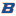Boise State University

An Exploration Of The Chromatic Polynomial, Amanda Aydelotte

In 1912, George Birkhoff was studying the Four Color Problem, and in doing so introduced the concept of the chromatic polynomial. While this did not end up directly contributing to proving that every map could be colored with four colors such that no region shares a border with another region of the same color, the chromatic polynomial has been found to have some very interesting properties. In this paper, it will be our goal to examine some of these properties and use them to determine information about their corresponding graphs.

Classification Of Minimal Separating Sets In Low Genus Surfaces, 2017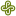Portland State University

Classification Of Minimal Separating Sets In Low Genus Surfaces, J. J. P. Veerman, William Maxwell, Victor Rielly, Austin K. Williams

Mathematics and Statistics Faculty Publications and Presentations

Consider a surface S and let MS. If S \ M is not connected, then we say M separates S, and we refer to M as a separating set of S. If M separates S, and no proper subset of M separates S, then we say M is a minimal separating set of S. In this paper we use computational methods of combinatorial topology to classify the minimal separating sets of the orientable surfaces of genus g = 2 and g = 3. The classification for genus 0 and 1 was done in earlier work, using methods of algebraic topology.

Optimal Layout For A Component Grid, 2017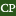California Polytechnic State University, San Luis Obispo

Optimal Layout For A Component Grid, Michael W. Ebert

Computer Science and Software Engineering

Several puzzle games include a specific type of optimization problem: given components that produce and consume different resources and a grid of squares, find the optimal way to place the components to maximize output. I developed a method to evaluate potential solutions quickly and automated the solving of the problem using a genetic algorithm.

Edge Colorings Of Complete Multipartite Graphs Forbidding Rainbow Cycles, 2017Auburn University

Edge Colorings Of Complete Multipartite Graphs Forbidding Rainbow Cycles, Peter Johnson, Andrew Owens

Theory and Applications of Graphs

It is well known that if the edges of a finite simple connected graph on n vertices are colored so that no cycle is rainbow, then no more than n-1 colors can appear on the edges. In previous work it has been shown that the essentially different rainbow-cycle-forbidding edge colorings of Kn with n-1 colors appearing are in 1-1 correspondence with (can be encoded by) the (isomorphism classes of) full binary trees with n leafs. In the encoding, the natural Huffman labeling of each tree arising from the assignment of 1 to each leaf plays a role. Very recently ...